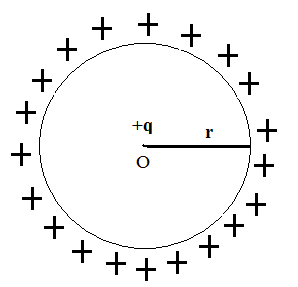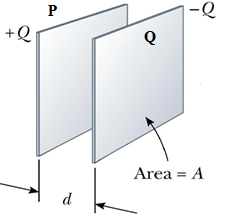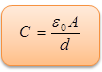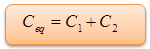×#### Thank you for registering.

One of our academic counsellors will contact you within 1 working day.

Click to Chat

1800-1023-196

+91-120-4616500

CART 0

• 0

MY CART (5)

Use Coupon: CART20 and get 20% off on all online Study Material

ITEM
DETAILS
MRP
DISCOUNT
FINAL PRICE
Total Price: Rs.

There are no items in this cart.
Continue Shopping• Complete Physics Course - Class 11
• OFFERED PRICE: Rs. 2,968
• View Details

Capacitors

Table of Content

Conductors

Capacity

Capacity of an Isolated Spherical Conductor

Principle of a Capacitor

Parallel Plate Capacitor

Effect of dielectric on the Capacitance of a Capacitor

Capacitors in Series

Cpacitors in Parallel

Related Resources

Conductors

Conductors are those substance through which electric charge easily.

Insulators: Insulators (also called dielectrics) are those substances through which electric charge cannot pass easily.Capacity

The potential V of a conductor depends upon the charge Q given to it. It has been observed that the potential of a conductor is proportional to the charge on it.

Q ∝V or Q = CV

The proportionality constant ‘C’ is known as the capacity of the conductor. Thus,

C = Q/V

The capacity of a conductor is defined as the ratio between the charge of the conductor to its potential.

If V = 1, then C = Q

The capacity of a conductor is also defined as the charge required to raise it through a unit potential.

Units

The capacity of a conductor is said to be 1 farad if a charge of 1 coulomb is required, to raise its potential through 1 volt.

The capacity of a conductor is said to be 1 stat farad is a charge of 1 stat coulomb is required, to raise its potential through 1 stat volt.

Dimension of C:- [M-1L-2T4A2]

Capacity of an Isolated Spherical ConductorConsider a spherical conductor of radius ‘r’ completely isolated from other charged bodies and situated in air. Let a charge ‘q’ be given to it. For calculation purposes, the charge ‘q’ can be supposed to be concentrated at the center of the sphere. The capacity of a conductor can be obtained as follows:

Charge on the sphere = q

Potential of the surface of the sphere = (1/4πε0r) (q/r)

Capacity, C = charge/potential = [q/(q/4πε0r)] = 4πε0r

But 1/4πε0r = 9109

So, 4πε0r = 1/9109

Thus, C = r/9109

Here, ‘C’ is in farad and ‘r’ is taken in meter.

A capacitor or a condenser is an arrangement which provides a larger capacity in a smaller space.

Principle of a Capacitor

It is based on the principle that when, an earthed conductor is placed in the neighborhood of a charged conductor, the capacity of the system increases considerably.

Parallel Plate CapacitorIt consists of two plates P and Q of a conducting material held parallel to each other, separated by a certain distance as shown in the figure. The space between the two plates contains some insulating material (may be air). The plate P is charged while the plate Q is connected to the earth.

Consider two parallel plates P and Q separated by a distance ‘d’. Plate P is charged with a charge +q while plate Q is earthed. A unit positive charge placed at a point A in between P and Q experiences a force σ/2ε0 due to P and σ/2ε0 due to Q. Both the forces are in same direction. Therefore, net intensity at A is

E = σ/2ε0+σ/2ε0 = σ/ε0

If ‘dV’ is the difference of potential between two closely situated points around A, then,

dV = -

As the unit positive charge on the point of a observation A moves away from Q,  and  are directed away from each other.

Thus,  = E dr cos 180 = – E dr

and dV = –(-E dr) = E dr

So,

Since E =  σ/ε0

Thus,

V = (σ/ε0)d

Therefore, surface density of charge, σ = q/A

Where ‘A’ is the common area of two plates.

V = qd/ε0A

Capacity, C = charge/potential = q/(qd/ε0A)

Or, C = q (ε0A/qd)

Or,This the formula for a capacity of a parallel plate condenser.

Effect of dielectric on the Capacitance of a CapacitorConsider a parallel plate condenser having a separation ‘d’ between its plates and with dielectric slab of the thickness ‘t’ in between them. Let K be the dielectric constant of the material of the slab.

Let the plate P be charged with a charge ‘+q’.

Surface density of charge on P is

σ = q/A, where A is its area.

Let the electric field in the free space between the plate be E and that inside the dielectric slab be E'.

The, E = σ/ε0 and E' = σ/ε0K

The potential difference V between the two poles P and Q of the capacitor is the work done in taking a unit positive charge from Q to P which is equivalent to the work done in taking a unit positive charge through a distance (d-t) in free space plus the work done in taking a unit positive charge through a dielectric of thickness ‘t’.

Hence, V = [σ/ε0](d – t) + [σ/ε0K] t = σ/ε0 [d-t+(t/K)]

Thus, C = σA/{σ/ε0[d-t+(t/K)]}            (Since, c = q/V)

Or, C = ε0A/[d-t+(t/K)]             …... (1)

In case, the space between P and Q is completely filled with the dielectric medium, then t = d.

Thus from equation (1), ε0A/[d-d+(d/K)] =  ε0KA/d                 …... (2)

Comparing equations (1) and (2), one concludes that dielectric thickness ‘d’ is equivalent to a free space of thickness d/K; where K is the dielectric constant.

Refer this video to know more about on, “capacitor and capacitance”

Capacitors in SeriesCapacitors are said to be connected in series if the second plate of one is connected with the first plate of the next and so on. This leaves the first plate of first capacitor and the second plate of the last capacitors free plates. Assuming, as seems reasonable, that these plates carry zero charge when zero potential difference is applied across the two capacitors, it follows that in the presence of a non-zero potential difference the charge +Q on the positive plate of capacitor 2 must be balanced by an equal and opposite charge -Q on the negative plate of capacitor 1. Since the negative plate of capacitor 1 carries a charge -Q, the positive plate must carry a charge +Q. Likewise, since the positive plate of capacitor 2 carries a charge +Q, the negative plate must carry a charge -Q. The net result is that both capacitors possess the same stored charge Q. The potential drops, V1 and V2, across the two capacitors are, in general, different. However, the sum of these drops equals the total potential drop V applied across the input and output wires: i.e., V = V1+V2. The equivalent capacitance of the pair of capacitors is again Ceq = Q/V. Thus,

1/Ceq = V/Q = (V1+V2)/Q = V1/Q + V2/Q

So,Thus, the reciprocal of the resultant capacity of a number of capacitors, connected in series, is equal to the sum of the reciprocals of their individual capacities.

Cpacitors in ParallelConsider two capacitors connected in parallel: i.e., with the positively charged plates connected to a common input'' wire, and the negatively charged plates attached to a common output'' wire--see in figure. What is the equivalent capacitance between the input and output wires? In this case, the potential difference V across the two capacitors is the same, and is equal to the potential difference between the input and output wires. The total charge Q, however, stored in the two capacitors is divided between the capacitors, since it must distribute itself such that the voltage across the two is the same. Since the capacitors may have different capacitances, C1 and C2, the charges Q1 and Q2 may also be different. The equivalent capacitance Ceq of the pair of capacitors is simply the ratio Q/V, where Q = Q1+Q2 is the total stored charge. It follows that

Ceq = Q/V =[Q1+Q2 /V] = Q1/V + Q2/V

So,The potential difference (V) across each of them is same.

Thus, the resultant capacity of a number of capacitors, connected in parallel, is equal to the sum of their individual capacitors.Capacitance is the ratio of charge to potential difference.

Charge resides on the surface of a conductor.

Condensers are said to be in parallel if each of them has same potential difference across its coatings.

Condensers are said to be in series if each of them has same charge on its coatings.

Capacitance always decreases when you move the plate apart.

Capacitance always increases when you put a dielectric (instead of air) between the plates.

The dielectric constant is the property of the dielectric material and varies from one material to another increasing the capacitance by a factor of k.

The dielectric provides mechanical support between the two plates allowing the plates to be closer together without touching.

Permittivity of the dielectric increases the capacitance.

The dielectric increases the maximum operating voltage compared to air.

Problem (JEE Main)

The plate separation in a parallel plate condenser is d and plate area is A. If it is changed to V volt and battery is disconnected then the work done in increasing the plate separation to 2d will be,

(a) (3/2) (ε0AV2/d)        (b) (ε0AV2/d)

(c)  (2ε0AV2/d)             (d) (ε0AV2/2d)

Solution:

We know that, q = CV = ((ε0A/d)V

After charging q will become constant.

W = Uf – Ui

=q2/2Cf – q2/2Ci

= q2/2 [1/Cf – 1/Ci]

= (ε0AV/d)2/2 [2d/ε0A – d/ε0A]

= ε0AV2/2d

From the above observation we conclude that, option (d) is correct.Question 1

A parallel plate capacitor has a capacitance of C farad. It area of the plates is doubled and the distance between them is half, the capacitance of the capacitor is

Question 2

A capacitor is connected to supply with switch and the switch is connected between capacitor and supply. Initially switch is open at time zero, and then switch is closed. Then how capacitor behaves at time t = 0+ ?

(a) short circuit.                         (b) open circuit.

(c) dielectric losses decreases.   (d) dielectric losses decreases.

Question 3

A p.d. of 300 V is applied across series combination of 3 μF and 9 μF capacitors. The charge on each capacitor is ___________________ μC.

(a) 675          (b) 3600

(c) 240          (d) 7.5

Question 4

A parallel plate capacitor with air as dielectric remains connected across a battery of emf 6 V. The charge on the capacitor in this condition is Q. If the separation between the plates is decreased by 10% in this condition

and sufficient time is allowed to attain steady state, the charge on the capacitor will be

(a) unchanged

(b) increased by 9%, approximately

(c) decreased by 9%, approximately

(d) increased by 11%, approximately

Question 5

When the plate area of a capacitor increases

(a) the capacitance decreases

(b) the capacitance increases

(c) the capacitance is unaffected

(d) the voltage it can withstand increases

Question 6

When the voltage across a capacitor is tripled, the stored charge

(a) triples                (b) is cut to one-third

(b) stays the same    (d) doublesQ.1
Q.2
Q.3
Q.4
Q.5
Q.6

c

a

a

d

b
a

Related Resources

You might like to refer Energy Stored in a Capacitor.

For getting an idea of the type of questions asked, refer the  Previous Year Question Papers.

To read more, Buy study material of Electrostatics comprising study notes, revision notes, video lectures, previous year solved questions etc. Also browse for more study materials on Physics here.### Course Features

• 101 Video Lectures
• Revision Notes
• Previous Year Papers
• Mind Map
• Study Planner
• NCERT Solutions
• Discussion Forum
• Test paper with Video Solution